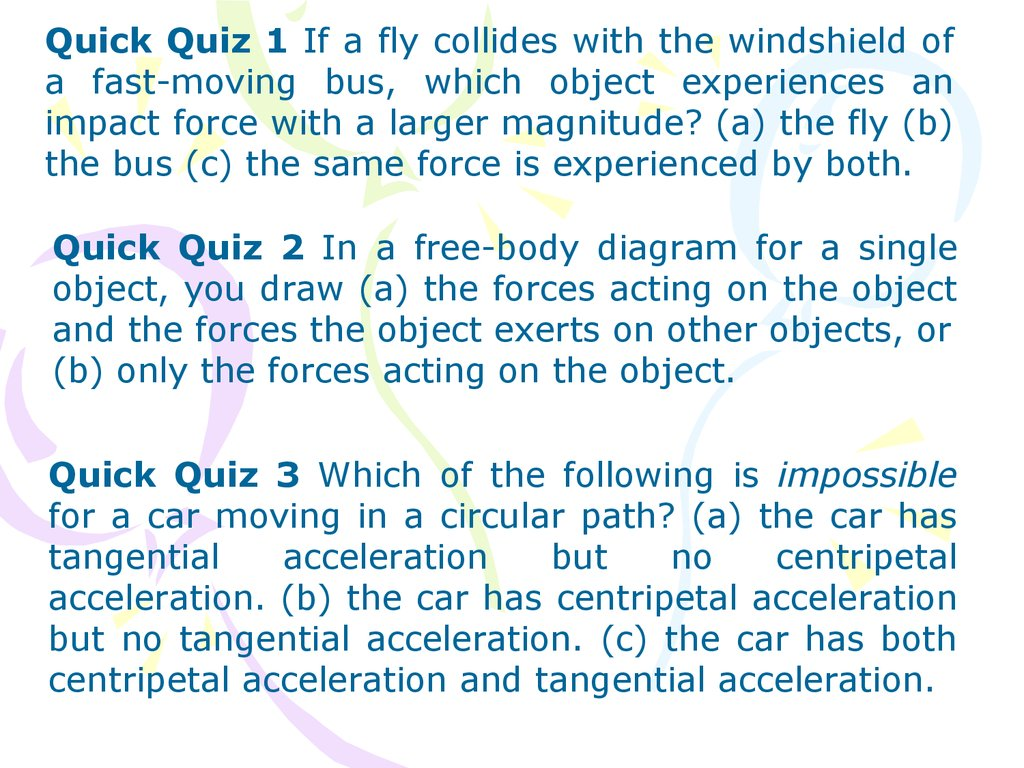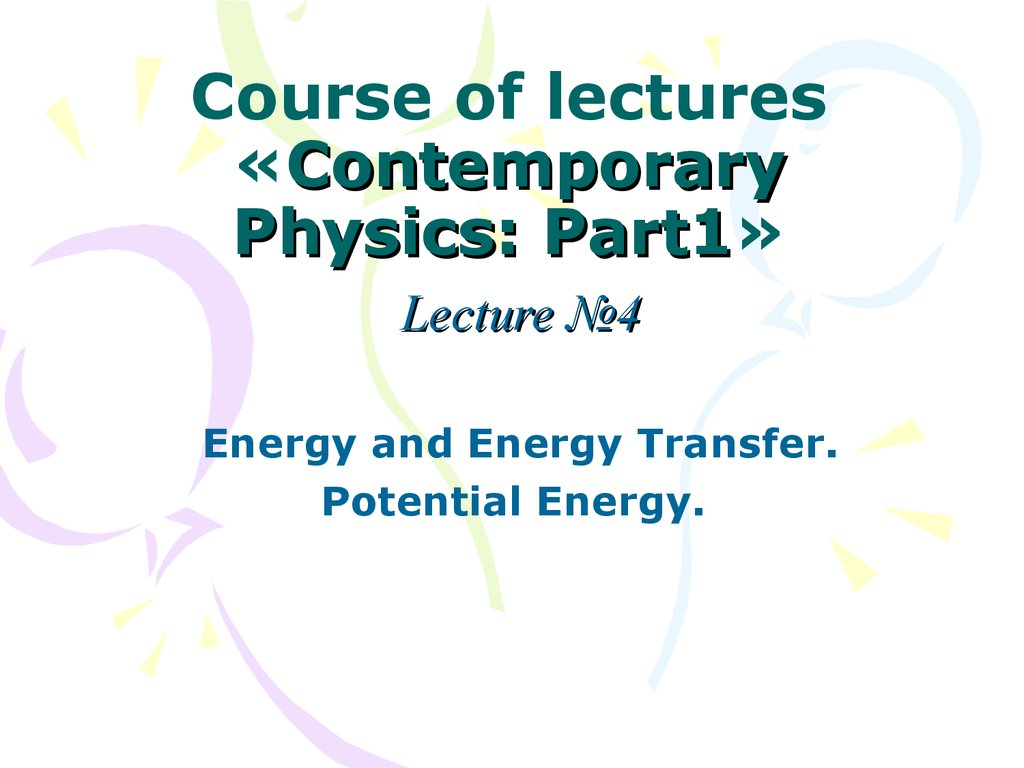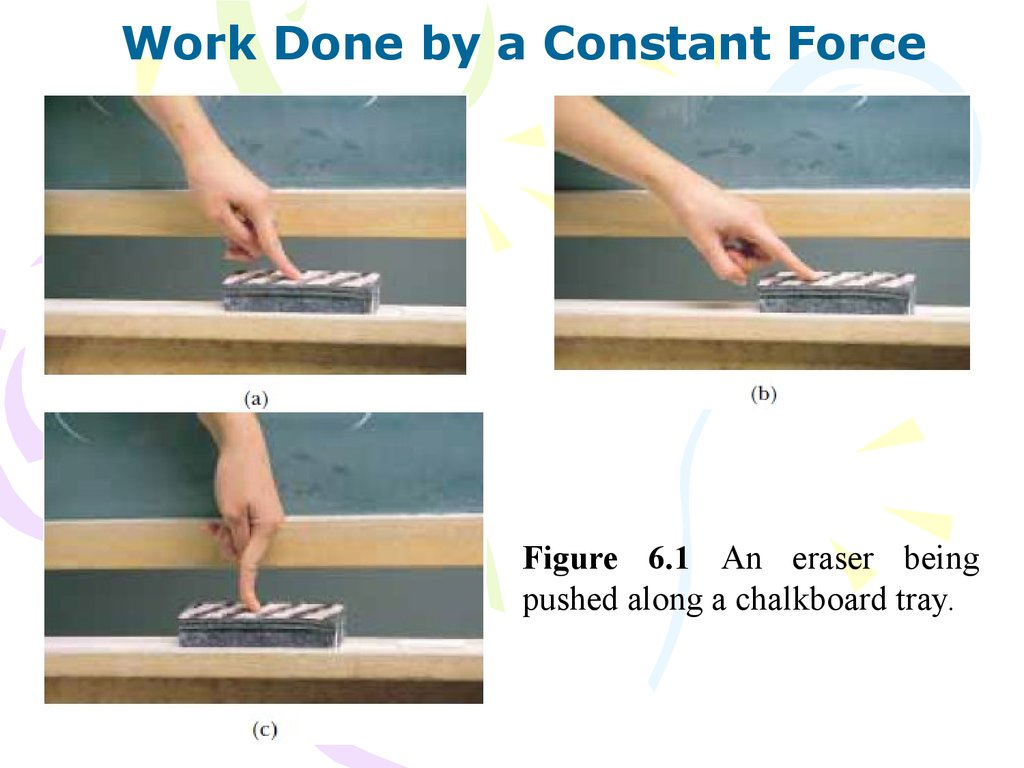# Quick Quiz

## 1.

Quick Quiz 1 If a fly collides with the windshield of
a fast-moving bus, which object experiences an
impact force with a larger magnitude? (a) the fly (b)
the bus (c) the same force is experienced by both.
Quick Quiz 2 In a free-body diagram for a single
object, you draw (a) the forces acting on the object
and the forces the object exerts on other objects, or
(b) only the forces acting on the object.
Quick Quiz 3 Which of the following is impossible
for a car moving in a circular path? (a) the car has
tangential
acceleration
but
no
centripetal
acceleration. (b) the car has centripetal acceleration
but no tangential acceleration. (c) the car has both
centripetal acceleration and tangential acceleration.

## 2. Course of lectures «Contemporary Physics: Part1»

Part1
Lecture №4
Energy and Energy Transfer.
Potential Energy.

## 3.

Work Done by a Constant Force
Figure 6.1 An eraser being
pushed along a chalkboard tray.

## 4.

Figure 6.2 If an
object undergoes a
displacement ∆r
under the action of
a constant force F,
the work done by
the
force
is
F∆rcosθ.
The work W done on a system by an agent exerting a constant force on
the system is the product of the magnitude F of the force, the
magnitude ∆ r of the displacement of the point of application of the
force, and cos θ, where θ is the angle between the force and
displacement vectors:
(6.1)

## 5.

Work is a scalar quantity, and
its units are force multiplied
by length. Therefore, the SI
unit of work is the newton·
meter
(N·m).
This
combination of units is used
so frequently that it has been
given a name of its own: the
joule ( J).
Figure 6.3 When an object is displaced on a frictionless, horizontal
surface, the normal force n and the gravitational force mg do no work
on the object. In the situation shown here, F is the only force doing
work on the object.

## 6.

An important consideration for a system
approach to problems is to note that work is an
energy transfer. If W is the work done on a
system and W is positive, energy is transferred
to the system; if W is negative, energy is
transferred from the system. Thus, if a system
interacts with its environment, this interaction
can be described as a transfer of energy across
the system boundary. This will result in a
change in the energy stored in the system.

## 7.

Work Done by a Varying Force
Figure 6.4 The work done by the force

## 8.

Figure 6.5 The work
done by the component
Fx of the varying force as
the particle moves from xi
to xf is exactly equal to
the area under this curve.
(6.2)
(6.3)

## 9.

Work Done by a Spring

## 10.

Kinetic Energy and the Work–
Kinetic Energy Theorem
(6.4)
Figure 6.6 An object undergoing a
displacement ∆r=∆xˆi and a change in
velocity under the action of a constant net
force ƩF.

## 11.

(6.5)
where vi is the speed of the block when it is at x = xi and vf is its
speed at xf.

## 12.

(6.6)
Kinetic energy is a scalar quantity and has the same units as work.
(6.7)
Equation 6.7 is an important result known as the work–kinetic energy
theorem:
In the case in which work is done on a system and the
only change in the system is in its speed, the work
done by the net force equals the change in kinetic
energy of the system.

## 13.

(a)
(c)
(b)
Figure
6.7
Energy
transfer
mechanisms. (a) Energy is transferred
to the block by work; (b) energy leaves
the radio from the speaker by
mechanical waves; (c) energy transfers
up the handle of the spoon by heat.

## 14.

(d)
(e)
(f)
Figure 6.7 Energy transfer
mechanisms. (d) energy enters
the automobile gas tank by
matter transfer; (e) energy
enters the hair dryer by
electrical transmission; and (f)
energy leaves the light bulb by

## 15.

One of the central features of the energy approach is the
notion that we can neither create nor destroy energy
—energy is always conserved. Thus, if the total
amount of energy in a system changes, it can only be
due to the fact that energy has crossed the boundary
of the system by a transfer mechanism such as one of
the methods listed above. This is a general statement
of the principle of conservation of energy. We can
describe this idea mathematically as follows:
(6.8)

## 16.

Power
The time rate of energy transfer is called power. If an
external force is applied to an object (which we
assume acts as a particle), and if the work done by this
force in the time interval ∆t is W, then the average
power during this interval is defined as

## 17.

In a manner similar to the way we approached the
definition of velocity and acceleration, we define the
instantaneous power as the limiting value of the average
power as ∆t approaches zero:
(6.9)

## 18.

In general, power is defined for any type of energy transfer.
Therefore, the most general expression for power is
(6.10)
The SI unit of power is joules per second ( J/s), also called the watt
(W) (after James Watt):
A unit of power in the U.S. customary system is the horsepower (hp):

## 19.

Potential Energy of a System
(6.11)
Figure 6.8 The work done by an
external agent on the system of the
book and the Earth as the book is
lifted from a height ya to a height yb
is equal to mgyb - mgya.

## 20.

The Isolated System–Conservation
of Mechanical Energy
Figure 6.9 The work done by the gravitational force on the book as the
book falls from yb to a height ya is equal to mgyb - mgya.

## 21.

Therefore, equating these two expressions for the work done on the
book,
(6.12)
Now, let us relate each side of this equation to the system of the book
and the Earth. For the right-hand side,
(6.13)
(6.14)

## 22.

We define the sum of kinetic and potential energies as mechanical
energy:
(6.15)
We will encounter other types of potential energy besides
gravitational later in the text, so we can write the general form of the
definition for mechanical energy without a subscript on U:
(6.16)
(6.17)

## 23.

(6.18)
Equation 6.18 is a statement of conservation of
mechanical energy for an isolated system. An
isolated system is one for which there are no
energy transfers across the boundary. The energy
in such a system is conserved—the sum of the
kinetic and potential energies remains constant.

## 24.

Conservative and
Nonconservative Forces
Conservative Forces
Nonconservative Forces

## 25.

Conservative forces have these two equivalent
properties:
1. The work done by a conservative force on a
particle moving between any two points is
independent of the path taken by the particle.
2. The work done by a conservative force on a
particle moving through any closed path is zero.
(A closed path is one in which the beginning and
end points are identical.)

## 26.

Nonconservative Forces
A force is nonconservative if it does not satisfy
properties 1 and 2 for conservative forces.
Nonconservative forces acting within a system cause
a change in the mechanical energy Emech of the system.
We have defined mechanical energy as the sum of the
kinetic and all potential energies.

## 27.

Changes in Mechanical Energy
for Nonconservative Forces
(6.19)
(6.20)

## 28.

Relationship Between Conservative Forces
and Potential Energy
(6.21)
(6.22)
(6.22)

## 29.

Relationship Between Conservative Forces
and Potential Energy
That is, the x component of a conservative force
acting on an object within a system equals the
negative derivative of the potential energy of the
system with respect to x.

## 30.

Quick Quiz 1 A block of mass m is projected across a horizontal
surface with an initial speed v. It slides until it stops due to the
friction force between the block and the surface. The same block
is now projected across the horizontal surface with an initial
speed 2v. When the block has come to rest, how does the
distance from the projection point compare to that in the first
case? (a) It is the same. (b) It is twice as large. (c) It is four
times as large. (d) The relationship cannot be determined.
Quick Quiz 2